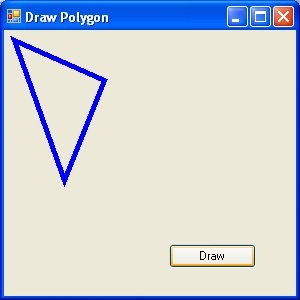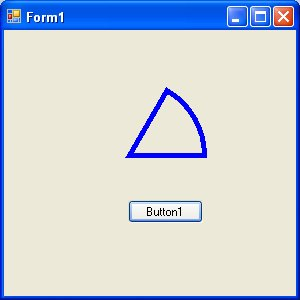# Visual Basic 2012 Lesson 25– Drawing Polygon and Pie

#### [Lesson 24] << [CONTENTS] >> [Lesson 26]

We have learned how to draw rectangle, ellipse, circle and text in the preceding chapters, now let’s learn how to draw polygons on the screen. Besides that, we shall also learn how to draw pie.

### 25.1: Drawing Polygons

Polygon is a closed plane figure bounded by three or more straight sides. In order to draw a polygon on the screen, we need to define the coordinates of all the points (also known as vertices) that joined up to form the polygon.

The syntax to defines the points of a polygon with vertices A1, A2, A3,  A4…….An is as follows;

```Dim A1 As New Point(X1,Y1)
Dim A2 As New Point(X2,Y2)
Dim A3 As New Point(X3,Y3)
Dim A4 As New Point(X4,Y4)
.
.
Dim An as New Point(Xn,Yn)
```

After declaring the points, we need to define a point structure that group all the points together using the following syntax:

`Dim myPoints As Point() = {A1, A2, A3,....,An}`

.Finally, create the graphics object and use the DrawPolygon method to draw the polygon using the following syntax:

```Dim myGraphics As Graphics = Me.CreateGraphics
myGraphics.DrawPolygon(myPen, myPoints)
```

where myPen is the Pen object created using the following syntax:

```myPen = New Pen(Drawing.Color.Blue, 5)
```

#### Example 25.1  Drawing a Triangle

A triangle is a polygon with three vertices. Here is the sample code:

```Dim myPen As Pen
Dim A As New Point(10, 10)
Dim B As New Point(100, 50)
Dim C As New Point(60, 150)
Dim myPoints As Point() = {A, B, C}
myPen = New Pen(Drawing.Color.Blue, 5)
Dim myGraphics As Graphics = Me.CreateGraphics
myGraphics.DrawPolygon(myPen, myPoints)
```

Running the program produces the image below:#### Example 25.2: Drawing a Quadrilateral

A quadrilateral is a polygon consist of four sides, so you need to define four vertices. The following is the code:

```Dim myPen As Pen
Dim A As New Point(10, 10)
Dim B As New Point(100, 50)
Dim C As New Point(120, 150)
Dim D As New Point(60, 200)
Dim myPoints As Point() = {A, B, C, D}
myPen = New Pen(Drawing.Color.Blue, 5)
Dim myGraphics As Graphics = Me.CreateGraphics
myGraphics.DrawPolygon(myPen, myPoints)
```

The output image is as shown below:### 25.2: Drawing Pie

In order to draw a pie, you can use the DrawPie method of the graphics object. As usual, you need to create the Graphics and the Pen objects. The syntax for drawing a pie is:

```myGraphics.DrawPie(myPen, X, Y, width,height, StartAngle, SweepAngle)
```

Where X and Y are the coordinates the bounding rectangle, other arguments are self-explanatory. Both StartAngle and SweepAngle are measured in degree. SweepAngle can take possible or negative values. If the value is positive,  sweeps through clockwise direction while negative means it sweep through anticlockwise direction.

#### Example 25.3

Drawing a pie that starts with 0 degrees and sweeps clockwise through 60 degrees.

```Dim myPen As Pen
myPen = New Pen(Drawing.Color.Blue, 5)
Dim myGraphics As Graphics = Me.CreateGraphics
myGraphics.DrawPie(myPen, 50,50, 150,150,0,60)
```

The output image is as shown below: